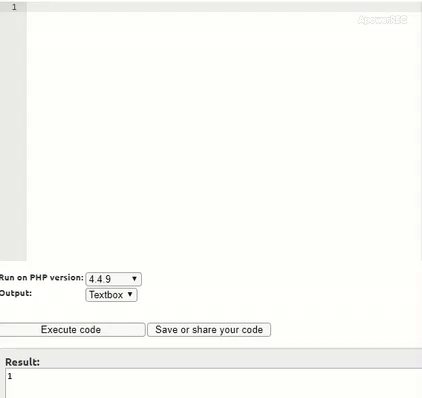# How to convert ereg expressions to preg in PHP ?

• Last Updated : 04 Aug, 2021

A regular expression is a specially formatted pattern that can be employed to find the instance of one string within another. There are various ways by which we can equip regular expressions to meet the requirement.

ereg() function: The new versions of PHP don’t support ereg anymore, so the outputs are given using the video when tried on an old version. One way being the use of ereg() function.

Examples:

• In this example, statement checks whether the subject provided to ereg() function is a string or not. Since the value given to compare ([a-zA-Z], starting from a to z and A to Z ) is a range. The ereg() function matches capital and small letters as provided in the range. The symbols have their own meaning.
```Input: echo ereg("[a-zA-Z]","jack");
Output: 1```
• This example checks whether the subject starts with ‘j’ or not. The symbol ‘^’ is used to check whether the subject starts with a required string or not.
```Input: echo ereg("^j","jack");
Output: 1```

preg() function: Another way of checking for regular expressions is by using preg() function. To use preg in your program it is advised to create a regex variable to compare with the subject. The following example provides the same functionality as the above code but by using preg.

Examples:

• Same as the ereg() function just using the preg function instead of ereg.
```Input:
\$regex='/[a-zA-Z]/';
echo preg_match(\$regex,'jack');
Output:1```
• This example is also the same as the second ereg example using just preg instead o ereg function.
```Input:
\$regex='/^[j]/';
echo preg_match(\$regex,"jack");
Output: 1```

Convert ereg expressions to preg: The above information depicts how ereg and preg work separately. The ereg and preg are generally worked the same but have a slight difference in the way they are employed. Now the answer to the question “how can you convert ereg to preg” is given below. The following steps can convert any ereg to preg.

• The statement which has to be used to compare with should be assigned to \$regex variable.
• Convert the double quotes (” “) to single ones (‘ ‘).
• The preg statements always start and end with backslash (‘\’).
• Both ereg and preg possess the same meaning for the symbols hence the logic remains the same.
• Both ereg and preg return boolean as an answer.

Example: This example implements how to convert ereg expressions to preg.

## PHP

 ``

Output:

`pregcheck at work : 1 eregcheck at work : 1 `My Personal Notes arrow_drop_up